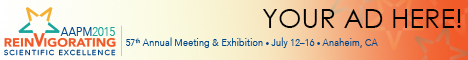Program Information

Separating Perfusion and Diffusion Components From Diffusion Weighted MRI of Rectum Tumors Based On Intravoxel Incoherent Motion (IVIM) AnalysisN Tyagi

Presentations

WE-G-18C-9 Wednesday 4:30PM - 6:00PM Room: 18C

Purpose: Pseudodiffusion arises from the microcirculation of blood in the randomly oriented capillary network and contributes to the signal decay acquired using a multi-b value diffusion weighted (DW)-MRI sequence. This effect is more significant at low b-values and should be properly accounted for in apparent diffusion coefficient (ADC) calculations. The purpose of this study was to separate perfusion and diffusion component based on a biexponential and a segmented monoexponential model using IVIM analysis.

Methods. The signal attenuation is modeled as S(b) = S0[(1-f)exp(-bD) + fexp(-bD*)]. Fitting the biexponetial decay leads to the quantification of D, the true diffusion coefficient, D*, the pseudodiffusion coefficient, and f, the perfusion fraction. A nonlinear least squares fit and two segmented monoexponential models were used to derive the values for D, D*,`and f. In the segmented approach b = 200 s/mm² was used as the cut-off value for calculation of D. DW-MRI’s of a rectum cancer patient were acquired before chemotherapy, before radiation therapy (RT), and 4 weeks into RT and were investigated as an example case.

Results: Mean ADC for the tumor drawn on the DWI cases was 0.93, 1.0 and 1.13 10⁻³x mm²/s before chemotherapy, before RT and 4 weeks into RT. The mean (D.10⁻³ x mm²/s, D* 10⁻³ x mm²/s , and f %) based on biexponential fit was (0.67, 18.6, and 27.2%), (0.72, 17.7, and 28.9%) and (0.83,15.1, and 30.7%) at these time points. The mean (D, D* f) based on segmented fit was (0.72, 10.5, and 12.1%), (0.72, 8.2, and 17.4%) and (.82, 8.1, 16.5% )

Conclusion: ADC values are typically higher than true diffusion coefficients. For tumors with significant perfusion effect, ADC should be analyzed at higher b-values or separated from the perfusion component. Biexponential fit overestimates the perfusion fraction because of increased sensitivity to noise at low b-values.

Contact Email: